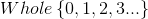# SAT II Math I : Number Sets

## Example Questions

### Example Question #1 : Number Sets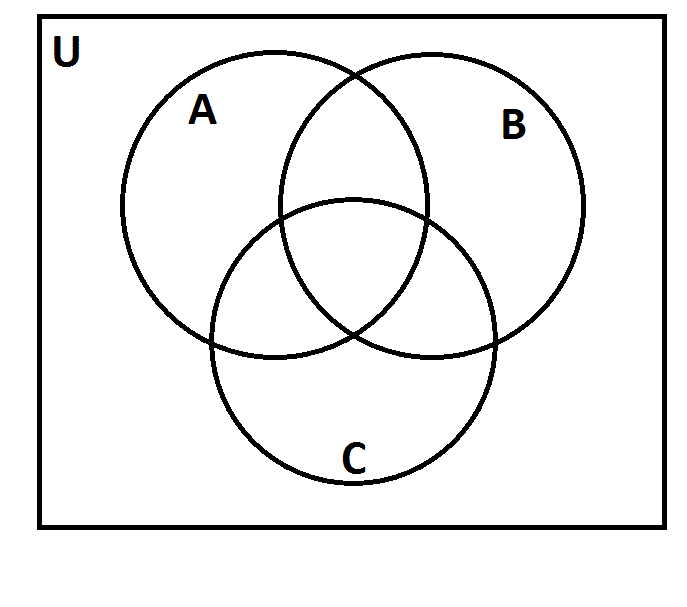The above represents a Venn diagram. The universal set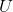is the set of all positive integers.

Letbe the set of all multiples of 2; letbe the set of all multiples of 3; letbe the set of all multiples of 5.

As you can see, the three sets divide the universal set into eight regions. Suppose each positive integer was placed in the correct region. Which of the following numbers would be in the same region as 450?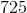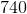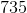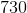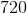Explanation:

450 ends in a "0" and is therefore a multiple of both 2 and 5. Also, since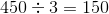,

450 is a multiple of 3.

Therefore,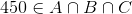.

We are looking for an element of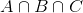- that is, a multiple of 2, 3, and 5. All five choices are multiples of 5 (ending in a "5" or a "0"); 725 and 735 can be eliminated as they are not multiples of 2 (ending in a "5"). We test 720, 730, and 740 to find the multiple of 3: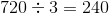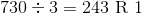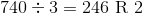720 is the correct choice.

### Example Question #2 : Number Sets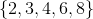Which of the above numbers does not fit with the rest of the set?Explanation:

In the above set, four of the numbers are all even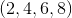.

The only one that is not even, also making it your answer, issince it is an odd number.

### Example Question #3 : Number Sets

Which pair of number sets have no intersection?

whole and natural

negative and even

integers and natural numbers

rational and irrational

prime and even

rational and irrational

Explanation:

An intersection of two sets is defined as the set of elements that are members of both sets. The correct answer is the pair of sets that has no overlap.

Rationals are defined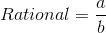if a and b are integers. The irrationals are defined as any real number that is not rational. By definition a number cannot be both rational and irrational.

Prime numbers are divisible only by 1 and themselves. Even numbers are defined as integer multiples of. A number common to both sets is.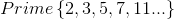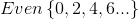Natural numbers are the counting numbers, while the integers are all the naturals and their opposites. There are many elements common to both sets, such as: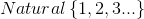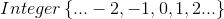The negatives are all the integers less than zero. And remember that an even number is defined as any integer multiple of two. Again there are many elements in common including: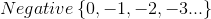The naturals are the counting numbers, while the wholes are all the naturals and zero. These two sets share many elements in common such as.